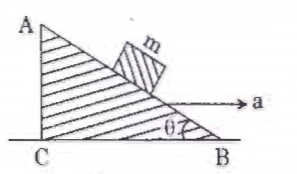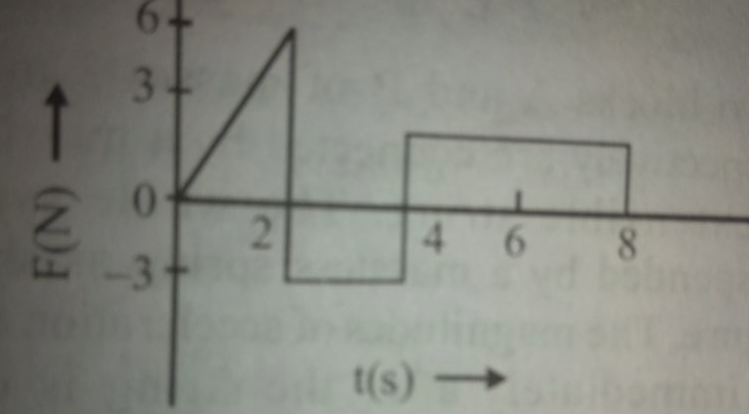## Filters

Sort by :
Clear All
Q

A particle moving with velocity  is acted by three forces shown by the vector triangle PQR . The velocity of the particle will :• Option 1)

increase

• Option 2)

derease

• Option 3)

remain constant

• Option 4)

change acording to the smallest force QR

Option 1) increase  Option 2) derease  Option 3) remain constant  Option 4) change acording to the smallest force QR

Two particle A abd B are moving in uniform circular motion in concentric  circles of radii  with speed  respectively . Their time period of rotation is the same . The ratio of angular speed of A to that of B will be :

• Option 1)

• Option 2)

• Option 3)

• Option 4)

1 : 1

A                B      as  Option 1)Option 2)Option 3)Option 4)1 : 1

A mass m is attached to a thin wire and whirled in a vertical circle  . The wire is most likely to break when :

• Option 1)

The mass is at the highest point

• Option 2)

the wire is horizontal

• Option 3)

The mass is at the lowest point

• Option 4)

Inclined  at an angle of 60 degree from vertical

The resultant of weight and centrifugal force is maximum at bottom . so wire is mostly like to break at bottom Option 1)The mass is at the highest point Option 2)the wire is horizontal Option 3)The mass is at the lowest point Option 4)Inclined  at an angle of 60 degree from vertical

A block of mass 10 Kg  is in contact against the inner wall of a hollow cylinder drum of radius 1 m . The coefficient of friction  between the block and the iner wall of the cylinder is 0.1 . The minimum angular velocity needed for the cylinder  to keep the block  stationary when the cylinder is vertical and rotating about its axis will be

• Option 1)

• Option 2)

• Option 3)

• Option 4)

Option 1) Option 2) Option 3) Option 4)
Here we have the FBD of the block on the wedge. By the figure,

A body of mass 1 kg begins to move
under the action of a time dependent force
(2ti + 3t2j)N, where i and j are unit
vectors along x and y axis. What power
will be developed by the force at the time

@gulamjilani

P=F.v

v is obtained by integrating acceleration

v=t^2i+t^3j

# A train 'A' runs from east to west and another train 'B' of the same mass runs from west to east at the same speed along the equator. 'A' presses the track with a force F1 and 'B' presses the track with a force F2

@ gulamjilani shaikh The earth rotates from west to east. If we view from the center of the earth, the trains will be performing Circular Motion. So, speed of the train that moves from west to east will be greater than that of the train moving from east to west; as, Speed of train moving from west to east=Speed of the train+wR and, Speed of train moving from east to west=Speed of the...

Screenshot_2019-03-05-13-17-01.pnga force F=(6i-8j+10k)N produces accelaration of 1m/s^2 in a body. Calculate mass of the body.

Mass of the body is:
jfjg.PNGA block of mass m is placed on a smooth inclined wedge ABC of inclination B as shown in the figure. The wedge is given an acceleration 'a' towards the right. The relation between theta and a for the block to reman stationary on the wedge is
@Krupa   For Block to be stationary net force along the plane =0
IMG-20190228-WA0000.jpg27. If a force F of 100 N is applied to block of mass 7 kg, then acceleration of 35 kg mass will be (g = 10 m/s2)
IMG_20190228_105106.jpg since force of static friction is< 100 N, body moves
Screenshot_632.pngTwo blocks of masses 50 Kg  and 30 Kg  connected by a massless string pass over a tight frictionless , pulley and rest on two smooth planes inclined at anglesnadreaspectively with horizontal as shown in the figure .If the system is released from rest then find time taken by 30 Kg block to reach the ground@Anupam here is the figure and solution     Adding (1)  and (2)  Now
IMG-20190110-WA0001.jpgThe pulleys and strings shown in figure are smooth and of negligible mass . for the system to remain in equilibrium , the angle theta should be
@Sudhir Bali From force diagram shown in figure,  T' =mg 2T'cosθ=2√mg So solving this we get    cosθ=1/√2 θ=45
A rope of length L and mass M hangs freely from the ceiling. If the time taken by a transverse wave to travel from the bottom to the top of the rope is T, then time to cover first half length is
T =  so for leth L/2 time period will be T/.
1548152985805-2009603303.jpg54.
In the given arrangement all surfaces are smooth. What acceleration should be given to the system, for which the
block m2 doesn't slide down?

@Aditya m1 and m2 doesn't slide down. means, m1 and m2 are rest with respect to M. now draw free body daigram for m1 and m2 in case of m1, T = m1a ........(1) in case of m2, T = m2g........(2) from eqs. (1) and (2),
1547444587624-1279057330.jpgA pulley is atttached to the ceiling of a lift moving upward. Two particles are attached to the two ends of a string passing over the pulley. The masses of the particles are in the ratio 2:1. If the acceleration of the particles is g/2 then acc of lift?
@Gourav Given  acceleration of the particles is g/2=a' now let acceleration of the lift=a upwards assume lift at rest and apply psudo force=mass* a to both masses Let masses as 2m and m 2m>m so 2m will move downward with a' So 2mg+2ma-T=2ma'=2m(g/2)=mg .....(1) and similarly for m mass T-mg-ma=ma'=m(g/2) .....(2) Solving both we get  a=(g/2)
A block is kept on an inclined plane of inclination theta & length l.The velocity of the particle at the bottom of inclined is@ Sayani Goswami  Acceleration  =g(sinθ−μ cosθ)  and s = l
Medical
95 Views   |

A block of mass m is placed on a smooth inclined wedge ABC of inclination   as shown in the figure. The wedge is given an acceleration 'a' towards the right. The relation between a and  for the block to remain stationary on the wedge is• Option 1)

• Option 2)

• Option 3)

• Option 4)

4) a =gtan

Medical
102 Views   |

Which one of the following statements is incorrect ?

• Option 1)

Frictional force opposes the relative motion.

• Option 2)

Limiting value of static friction is directly proportional to normal reaction.

• Option 3)

Rolling friction is smaller than sliding friction.

• Option 4)

Coefficient    of sliding friction    has
dimensions of length.

As we have learned Kinetic or Dynamic Friction -  kinetic friction   coefficient of kinetic friction R = reaction - wherein depends on the nature of surface in contact.    Coefficient of sliding friction is dimension lessThis is incorrect Option 1)  Frictional force opposes the relative motion. This is incorrect Option 2)  Limiting value of static friction is directly proportional to...
Medical
294 Views   |

Two stones of masses m and 2m are whirled in horizontal circles, the heavier one in radius  and the lighter one in radius r. The tangential speed of lighter stone is n times that of the  value of heavier stone when they experience same centripetal forces. The value of n is:

• Option 1)

3

• Option 2)

4

• Option 3)

1

• Option 4)

2

As we discussed in concept Force in non-uniform Circular Motion - Ft = mat m = mass ac= centripetal acceleration at = tangen tial acceleration Fc = centripetal force - wherein W is not equal to zero by tangential force. W = 0 by centripetal force         i.e n=2   Option 1) 3 This option is incorrect. Option 2) 4 This option is incorrect. Option 3) 1 This option is incorrect. Option...
Medical
228 Views   |

The force 'F' acting on a particle fo mass 'm' is indicated by the force-time graph shown below. The change in momentum of the particle over the time interval from zero to 8 s is:• Option 1)

24 Ns

• Option 2)

20Ns

• Option 3)

12Ns

• Option 4)

6Ns

As we discussed in concept Impulse - - wherein    Change in momentum Area of - area of rectange + area of rectange   Option 1) 24 Ns This option is incorrect. Option 2) 20Ns This option is incorrect. Option 3) 12Ns This option is correct. Option 4) 6Ns This option is incorrect.
Exams
Articles
Questions